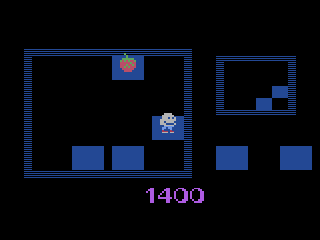n e w s a b o u t g a m e s p r o g r a m m e r s

 a t a r i . 2 6 0 0 c l i m b e r 5 g u n f i g h t j a m m e d m a r b l e c r a z e o y s t r o n q b s p a c e i n s t i g a t o r s s t a r f i r e t h r u s t

 c o l e c o v i s i o n s p a c e i n v a d e r s c o l l e c t i o np a c k

 v i d e o p a c / o d y s s e y 2 k i l lt h ea t t a c k i n ga l i e n s

 q bBy Andrew Davie
Cartridges available at Atariage.com